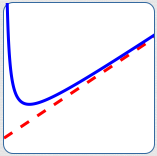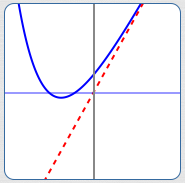﻿ Finding Slant Asymptotes

#Finding Slant Asymptotes

Drop an object from far above the Earth, and it falls faster and faster—for a while.

Indeed, since acceleration due to gravity is about $\frac{9.8 \text{ meters per second}}{\text{second}}\,,$ its speed initially increases by about 9.8 m/sec every second.

But, it doesn't keep going faster and faster. Due to frictional forces, it settles on a so-called ‘terminal velocity’.

If you model distance traveled versus time mathematically, you'll be looking at a slant asymptote.

Functions can ‘get big’ in different ways. When they ‘get big’ by looking more and more like a ‘slanted’ line (i.e., not horizontal and not vertical), then the function is said to have a slant asymptote.

Slant asymptotes are also called oblique asymptotes.

As discussed in Introduction to Asymptotes, an asymptote is a curve (usually a line) that another curve gets arbitrarily close to as $\,x\,$ approaches $\,+\infty\,,$ $\,-\infty\,,$ or a finite number.

Here's how this general definition is ‘specialized’ to get a slant asymptote:

A ‘slant asymptote’ is a non-vertical, non-horizontal line that another curve gets arbitrarily close to as $\,x\,$ approaches $\,+\infty\,$ or $\,-\infty\,.$ (See image below.)

What's the key to a slant asymptote situation?

The outputs are behaving more and more like $\,y = mx + b\,$ for $\,m\ne 0\,$ as inputs get arbitrarily large (big and positive, or big and negative).

This section gives a precise discussion of slant asymptotes, as well as conditions under which a rational function has a slant asymptote.The red line is a slant asymptote for the blue curve.

As $\,x\rightarrow\infty\,,$ the blue curve approaches the red line.

Conditions under which $\,y = mx + b\,$ ($\,m\ne 0\,$) is a Slant Asymptote for a Function $\,f$

Let $\,f\,$ be a nonlinear function. That is, $\,f(x)\,$ is not of the form $\,f(x) = cx + d\,$ for any real numbers $\,c\,$ and $\,d\,.$

Let $\,m \ne 0\,.$ The line $\,y = mx + b\,$ is a slant asymptote for $\,f\,$ if and only if at least one of the following conditions holds:

• As $\,x\rightarrow\infty\,,$ $\,f(x) - (mx + b) \rightarrow 0\,$
• As $\,x\rightarrow -\infty\,,$ $\,f(x) - (mx + b) \rightarrow 0\,$

That is, the vertical distance between the line $\,y = mx + b\,$ and the graph of $\,f\,$ approaches zero as $\,x\rightarrow\infty\,$ or $\,x\rightarrow -\infty\,$ (or both).

• A function can have at most two slant asymptotes. It can approach one line as $\,x\rightarrow\infty\,,$ and a different line as $\,x\rightarrow -\infty\,.$
• A function can have a slant asymptote and a horizontal asymptote. For example, it might approach a horizontal line as $\,x\rightarrow\infty\,,$ and a slanted line as $\,x\rightarrow -\infty\,.$

## A Non-Rational Function With a Slant Asymptote

Let $\,f(x) = 3x + \text{e}^{-x}\,$ (the blue curve below).As $\,x\rightarrow \infty\,,$ we have $\,\text{e}^{-x}\rightarrow 0\,,$ so the outputs from $\,f\,$ are looking more and more like $\,3x\,.$

More precisely, as $\,x\rightarrow \infty\,,$ we have:

$$\begin{gather} \cssId{s41}{f(x) - 3x \ = \ (3x + \text{e}^{-x}) - 3x \ = \ \text{e}^{-x}}\cr \cssId{s42}{\text{and}}\cr \cssId{s43}{\text{e}^{-x}\rightarrow 0} \end{gather}$$

Thus, $\,y = 3x\,$ (the red line above) is a slant asymptote for $\,f\,.$

## A Rational Function With a Slant Asymptote: Degree of Numerator is Exactly One Greater Than Degree of Denominator

Let $\displaystyle\,R(x) = \frac{x^2 - 4x - 5}{x - 3}\,.$

When $\,x\,$ is big, the outputs from $\,R(x)\,$ look like $\,\frac{\text{big}}{\text{big}}\,,$ which is not very useful. As usual, we will rename the function to better understand what happens when $\,x\,$ is big.

The key is to do a long division:

 $x$ $-$ $1$ $x-3$ $x^2$ $-$ $4x$ $-$ $5$ $-(x^2$ $-$ $3x$ $)$ $-x$ $-$ $5$ $-($ $-x$ $+$ $3)$ $-8$

Thus:

\begin{align} \cssId{s54}{R(x)}\ &\cssId{s55}{= \frac{x^2 - 4x - 5}{x - 3}}\cr &\cssId{s56}{=\ x - 1 \ \ \ + \overbrace{\left(\frac{-8}{x-3}\right)}^{\text{this part tends to zero}}} \end{align}

Note that as $\,x\rightarrow\pm\infty\,,$  $\displaystyle\,\frac{-8}{x-3}\rightarrow 0\,.$

Therefore, as inputs get big, the function is looking more and more like the line $\,y = x - 1\,.$ Thus, $\,y = x - 1\,$ is a slant asymptote for the function $\,R\,.$

The graph of the function $\,\color{blue}{R}\,$ (in blue), together with its slant asymptote $\,\color{red}{y = x - 1}\,$ (in red), is shown below.When we were doing the long division of $\,x^2 - 4x - 5\,$ by $\,x - 3\,$ up above, what caused the $\,x = x^1\,$ to appear in the quotient $\,x - 1\,$?

It was the fact that the degree of the numerator is one more than the degree of the denominator! This is the key ingredient for determining if a rational function has a slant asymptote. (There are a couple other technical requirements, which are stated below.)

In summary, we have:

Conditions under which a Rational Function has a Slant Asymptote

Let $\,N(x)\,$ and $\,D(x)\,$ be polynomials, so that $\displaystyle\,R(x) = \frac{N(x)}{D(x)}\,$ is a rational function.

The function $\,R\,$ has a slant asymptote when the following conditions are met:

• $\deg{N(x)} = \deg{D(x)} + 1$
(The degree of the numerator is exactly one more than the degree of the denominator.)
• $\deg{N(x)} \ge 2$
(The numerator is at least quadratic.)
• When dividing $\,D(x)\,$ into $\,N(x)\,,$ the remainder is not zero.

### Why Do We Require That $\deg{N(x)}\ge 2\,$?

Notice that $\displaystyle \,y = \frac{x-1}{3}\,$ is a rational function, since both numerator and denominator are polynomials.

The degree of the numerator is $\,1\,.$ The degree of the denominator is $\,0\,.$ Thus, the degree of the numerator is exactly one more than the degree of the denominator.

However, this is just the linear function $\,y = \frac 13x - \frac 13\,,$ which does not have a slant asymptote.

### Why Do We Require The Remainder To Be Nonzero?

Notice that $\displaystyle \,y = \frac{x^2-1}{x-1}\,$ is a rational function, and the degree of the numerator is exactly one more than the degree of the denominator.

However, in this case $\,x - 1\,$ goes into $\,x^2 - 1\,$ evenly (the remainder is zero):

\begin{align} y \ \ &\cssId{s87}{= \frac{x^2-1}{x-1}}\cr\cr &\cssId{s88}{= \frac{(x-1)(x+1)}{x-1}}\cr\cr &\cssId{s89}{= x + 1\,,} \ \ \cssId{s90}{\text{for \,x\ne 1\,}} \end{align}

This function graphs as a line that is punctured at $\,x = 1\,.$ It does not have a slant asymptote.